# Chapter 6 Stacks Data Structures Using Java 1

• Slides: 48Chapter 6 Stacks Data Structures Using Java 1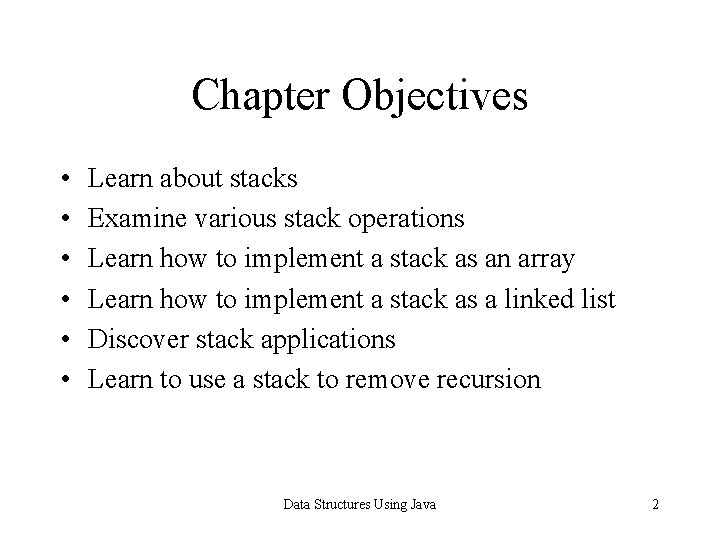Chapter Objectives • • • Learn about stacks Examine various stack operations Learn how to implement a stack as an array Learn how to implement a stack as a linked list Discover stack applications Learn to use a stack to remove recursion Data Structures Using Java 2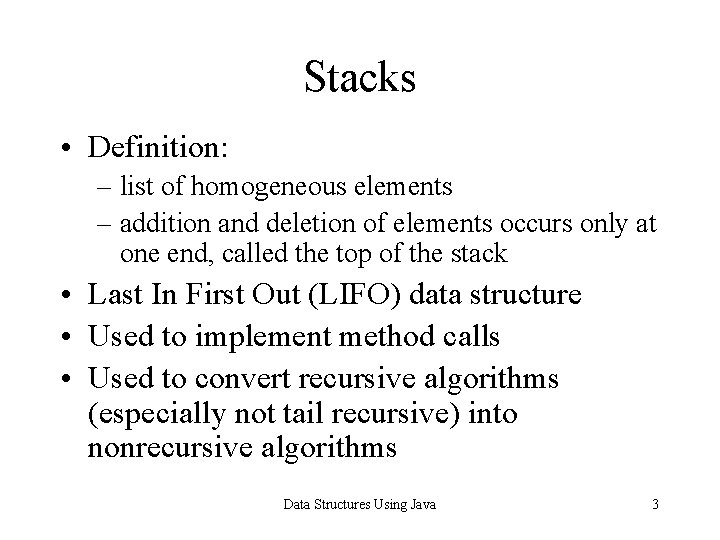Stacks • Definition: – list of homogeneous elements – addition and deletion of elements occurs only at one end, called the top of the stack • Last In First Out (LIFO) data structure • Used to implement method calls • Used to convert recursive algorithms (especially not tail recursive) into nonrecursive algorithms Data Structures Using Java 3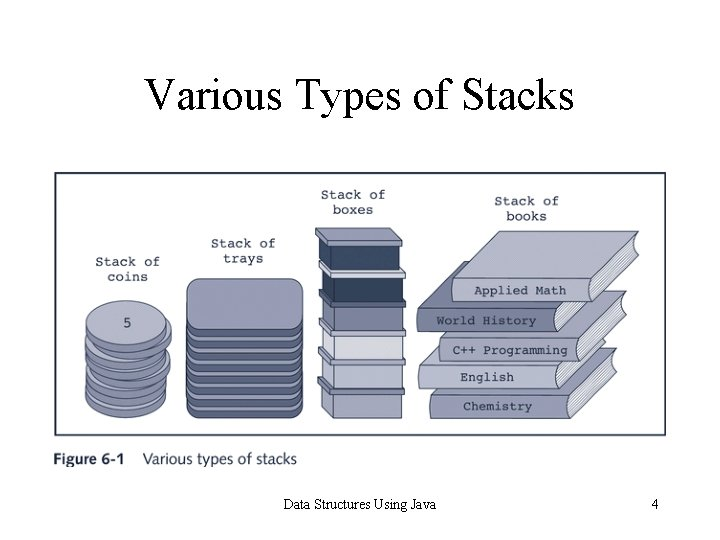Various Types of Stacks Data Structures Using Java 4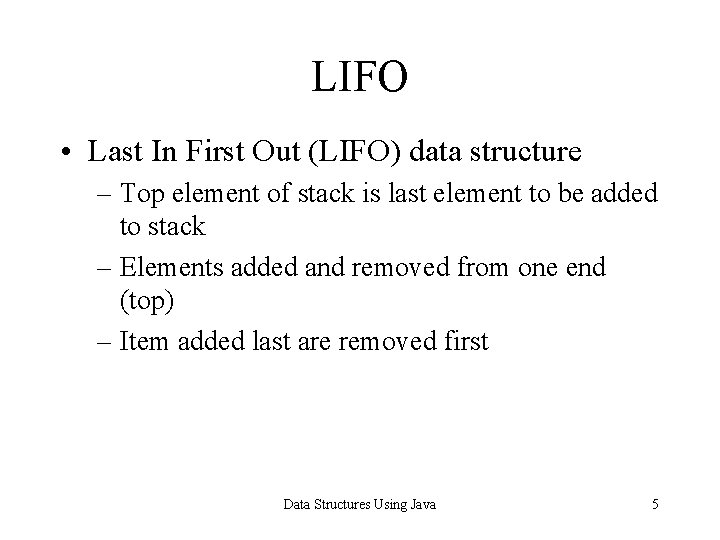LIFO • Last In First Out (LIFO) data structure – Top element of stack is last element to be added to stack – Elements added and removed from one end (top) – Item added last are removed first Data Structures Using Java 5Empty Stack Data Structures Using Java 6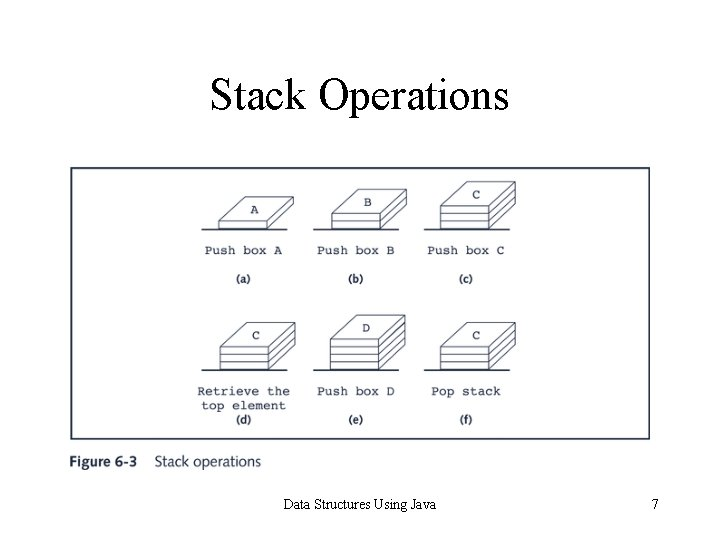Stack Operations Data Structures Using Java 7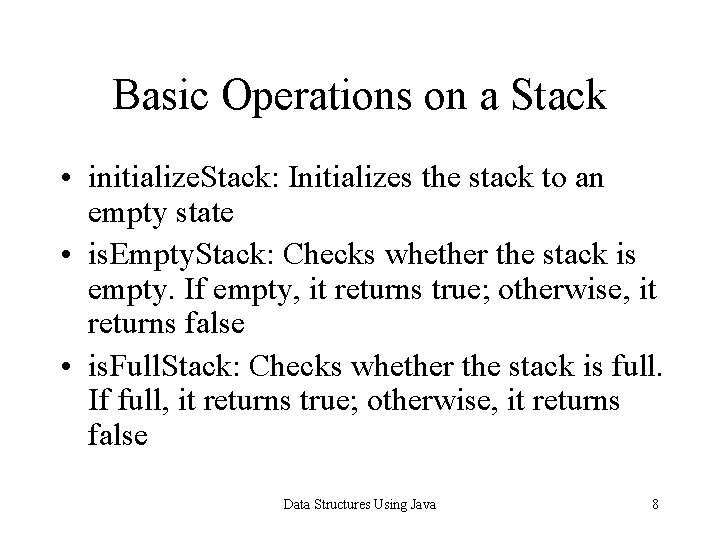Basic Operations on a Stack • initialize. Stack: Initializes the stack to an empty state • is. Empty. Stack: Checks whether the stack is empty. If empty, it returns true; otherwise, it returns false • is. Full. Stack: Checks whether the stack is full. If full, it returns true; otherwise, it returns false Data Structures Using Java 8Basic Operations on a Stack • push: – Add new element to the top of the stack – The input consists of the stack and the new element – Prior to this operation, the stack must exist and must not be full Data Structures Using Java 9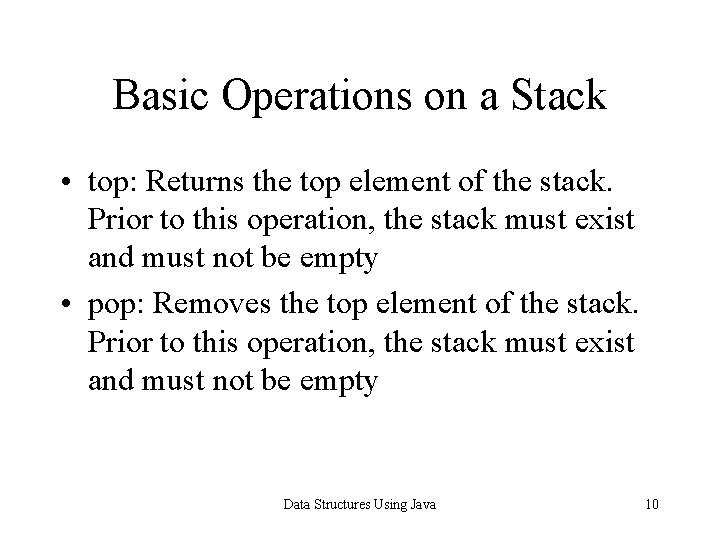Basic Operations on a Stack • top: Returns the top element of the stack. Prior to this operation, the stack must exist and must not be empty • pop: Removes the top element of the stack. Prior to this operation, the stack must exist and must not be empty Data Structures Using Java 10Example of a Stack Data Structures Using Java 11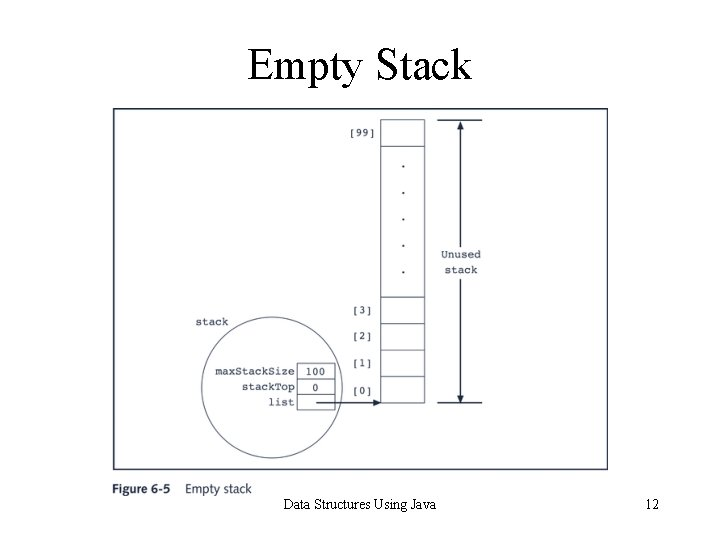Empty Stack Data Structures Using Java 12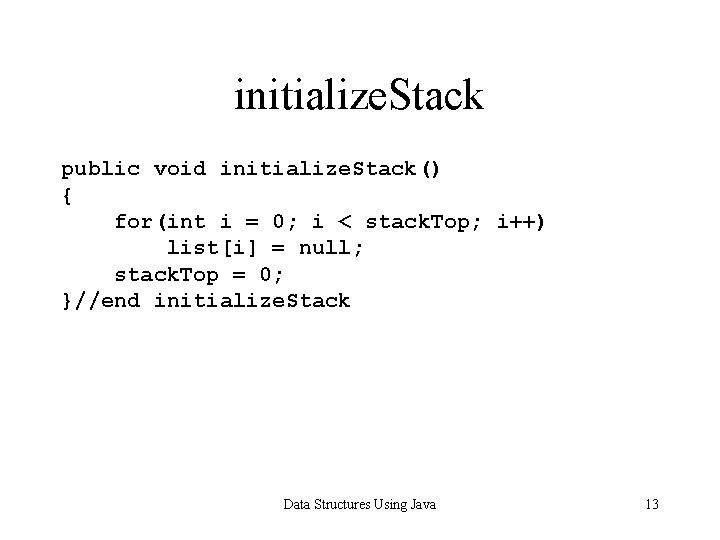initialize. Stack public void initialize. Stack() { for(int i = 0; i < stack. Top; i++) list[i] = null; stack. Top = 0; }//end initialize. Stack Data Structures Using Java 13empty. Stack and full. Stack public boolean is. Empty. Stack() { return(stack. Top == 0); }//end is. Empty. Stack public boolean is. Full. Stack() { return(stack. Top == max. Stack. Size); }//end is. Full. Stack Data Structures Using Java 14Push Data Structures Using Java 15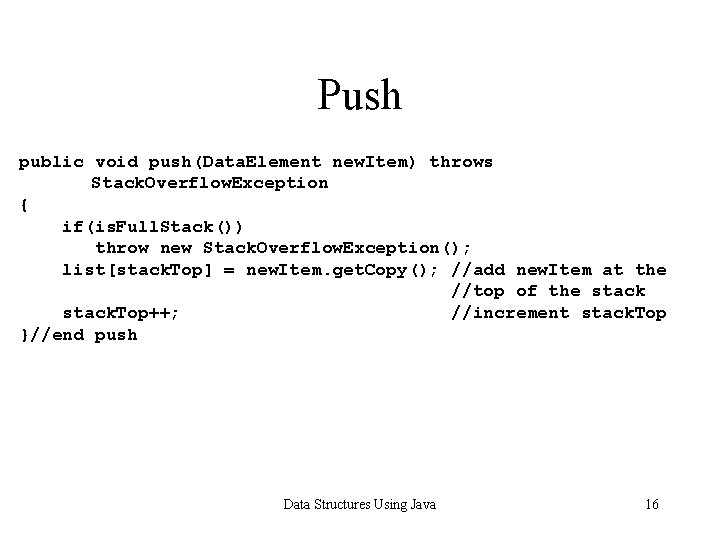Push public void push(Data. Element new. Item) throws Stack. Overflow. Exception { if(is. Full. Stack()) throw new Stack. Overflow. Exception(); list[stack. Top] = new. Item. get. Copy(); //add new. Item at the //top of the stack. Top++; //increment stack. Top }//end push Data Structures Using Java 16Return Top Element public Data. Element top() throws Stack. Underflow. Exception { if(is. Empty. Stack()) throw new Stack. Underflow. Exception(); Data. Element temp = list[stack. Top - 1]. get. Copy(); return temp; }//end top Data Structures Using Java 17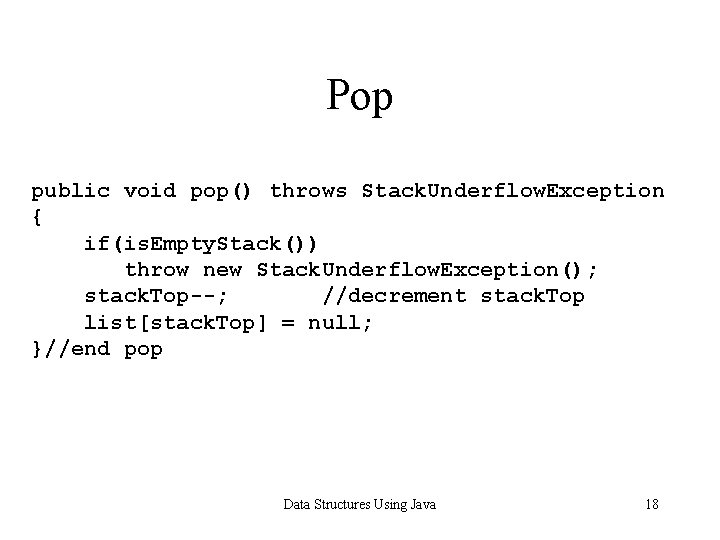Pop public void pop() throws Stack. Underflow. Exception { if(is. Empty. Stack()) throw new Stack. Underflow. Exception(); stack. Top--; //decrement stack. Top list[stack. Top] = null; }//end pop Data Structures Using Java 18Pop Data Structures Using Java 19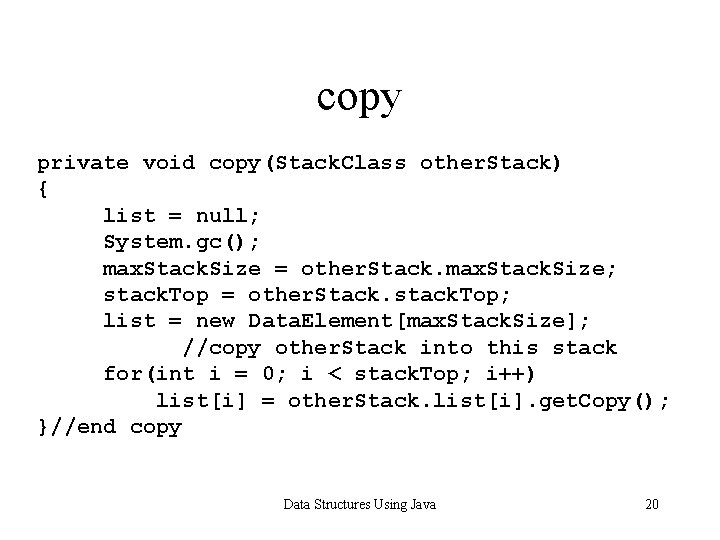copy private void copy(Stack. Class other. Stack) { list = null; System. gc(); max. Stack. Size = other. Stack. max. Stack. Size; stack. Top = other. Stack. stack. Top; list = new Data. Element[max. Stack. Size]; //copy other. Stack into this stack for(int i = 0; i < stack. Top; i++) list[i] = other. Stack. list[i]. get. Copy(); }//end copy 6 Data Structures Using Java 20Constructors //constructor with a parameter public Stack. Class(int stack. Size) { if(stack. Size <= 0) { System. err. println(“The size of the array to implement “ + “the stack must be positive. ”); System. err. println(“Creating an array of size 100. ”); max. Stack. Size = 100; } else max. Stack. Size = stack. Size; //set the stack size to //the value specified by //the parameter stack. Size stack. Top = 0; //set stack. Top to 0 list = new Data. Element[max. Stack. Size]; //create the array }//end constructor Data Structures Using Java 21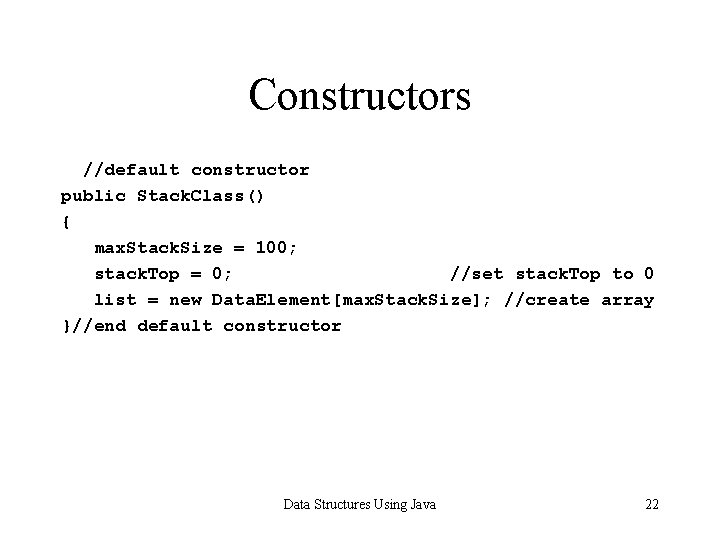Constructors //default constructor public Stack. Class() { max. Stack. Size = 100; stack. Top = 0; //set stack. Top to 0 list = new Data. Element[max. Stack. Size]; //create array }//end default constructor Data Structures Using Java 22Copy Constructor and copy. Stack public Stack. Class(Stack. Class other. Stack) { copy(other. Stack); }//end copy constructor public void copy. Stack(Stack. Class other. Stack) { if(this != other. Stack) //avoid self-copy(other. Stack); }//end copy. Stack Data Structures Using Java 23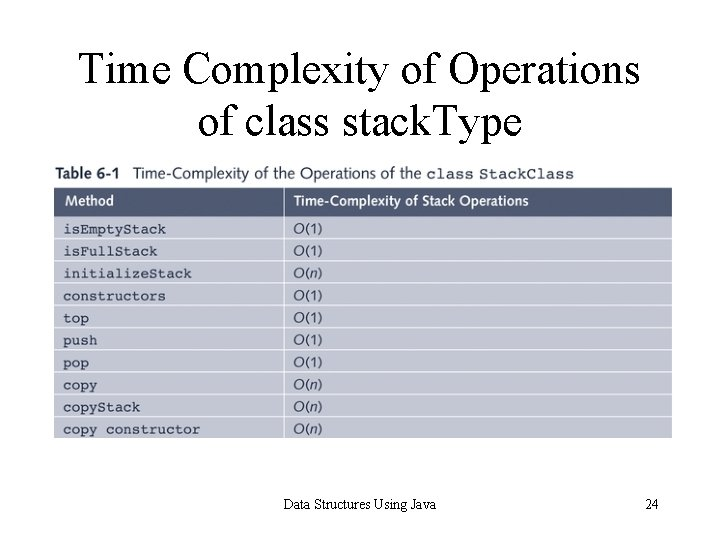Time Complexity of Operations of class stack. Type Data Structures Using Java 24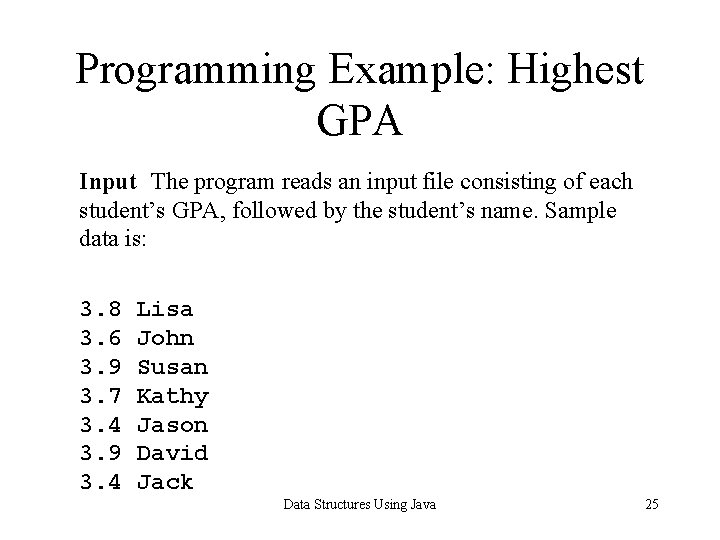Programming Example: Highest GPA Input The program reads an input file consisting of each student’s GPA, followed by the student’s name. Sample data is: 3. 8 3. 6 3. 9 3. 7 3. 4 3. 9 3. 4 Lisa John Susan Kathy Jason David Jack Data Structures Using Java 25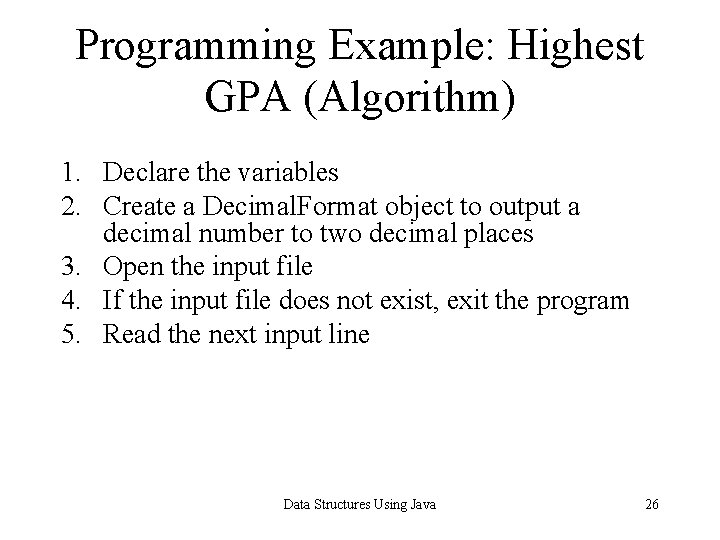Programming Example: Highest GPA (Algorithm) 1. Declare the variables 2. Create a Decimal. Format object to output a decimal number to two decimal places 3. Open the input file 4. If the input file does not exist, exit the program 5. Read the next input line Data Structures Using Java 26Highest GPA (Algorithm) 6. while (not end of file) { 6. a. Tokenize the input line 6. b. Get the next GPA 6. c. Get the next name 6. d. if (GPA > highest. GPA) { 6. d. i initialize stack 6. d. ii push(stack, student name) 6. d. iii highest. GPA = GPA } 6. e. else if(GPA is equal to highest. GPA) push(stack, student name) 6. f Read the next input line } Data Structures Using Java 27Programming Example: Highest GPA (Algorithm) 7. 8. Output the highest GPA. Output the names of the students having the highest GPA. Data Structures Using Java 28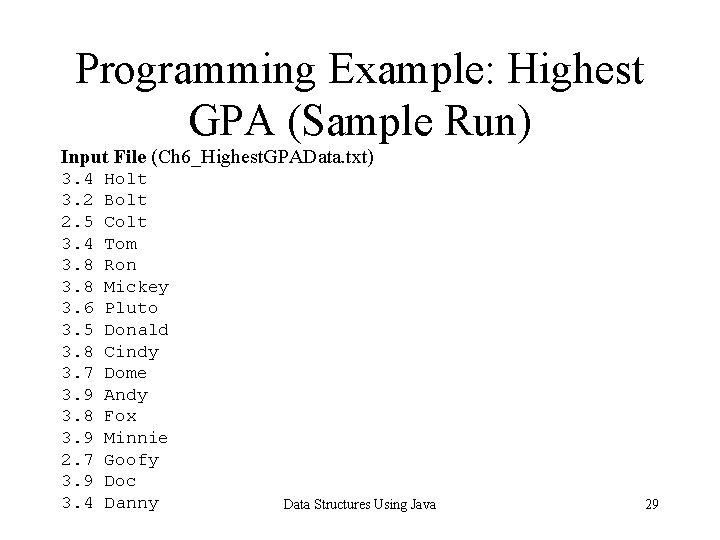Programming Example: Highest GPA (Sample Run) Input File (Ch 6_Highest. GPAData. txt) 3. 4 3. 2 2. 5 3. 4 3. 8 3. 6 3. 5 3. 8 3. 7 3. 9 3. 8 3. 9 2. 7 3. 9 3. 4 Holt Bolt Colt Tom Ron Mickey Pluto Donald Cindy Dome Andy Fox Minnie Goofy Doc Danny Data Structures Using Java 29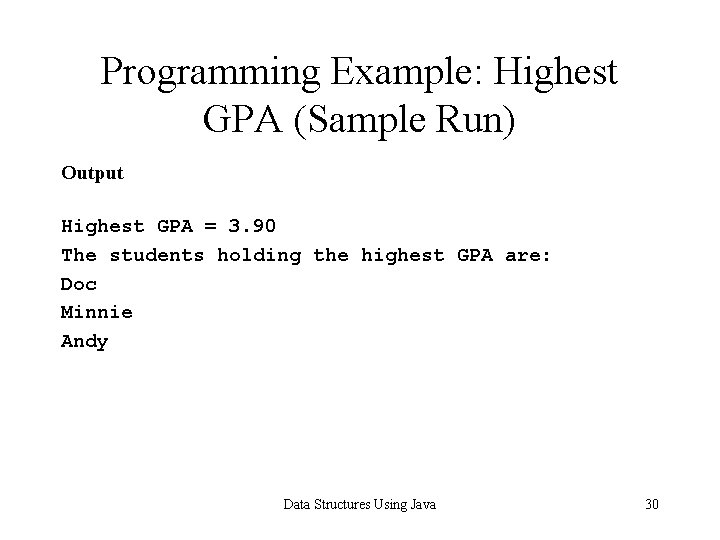Programming Example: Highest GPA (Sample Run) Output Highest GPA = 3. 90 The students holding the highest GPA are: Doc Minnie Andy Data Structures Using Java 30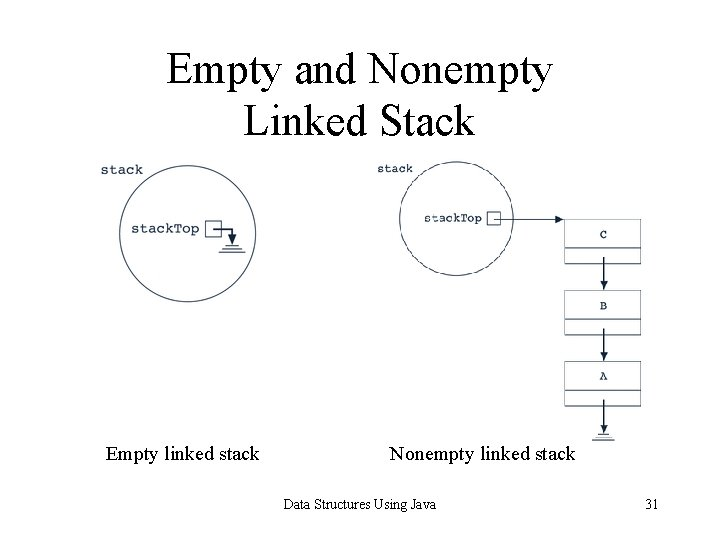Default Constructor public Linked. Stack. Class() { stack. Top = null; } Data Structures Using Java 32initialize. Stack, is. Stack. Empty, and is. Stack. Full public void initialize. Stack() { stack. Top = null; }//end initialize. Stack public boolean is. Empty. Stack() { return(stack. Top == null); } public boolean is. Full. Stack() { return false; } Data Structures Using Java 33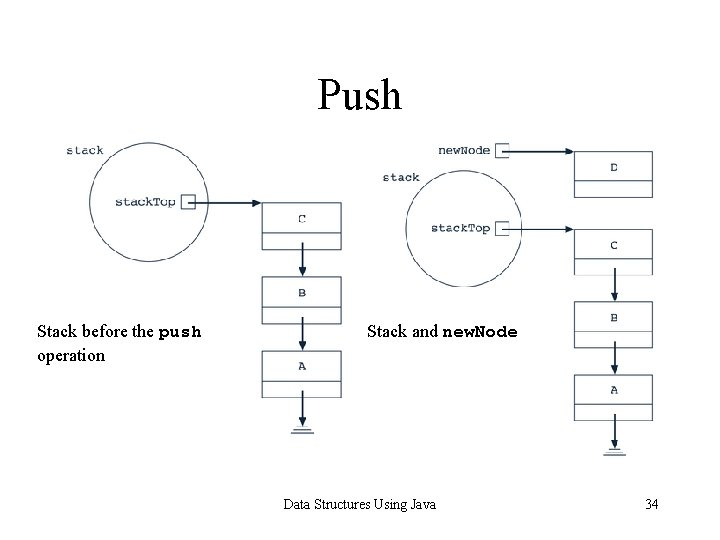Push Stack before the push operation Stack and new. Node Data Structures Using Java 34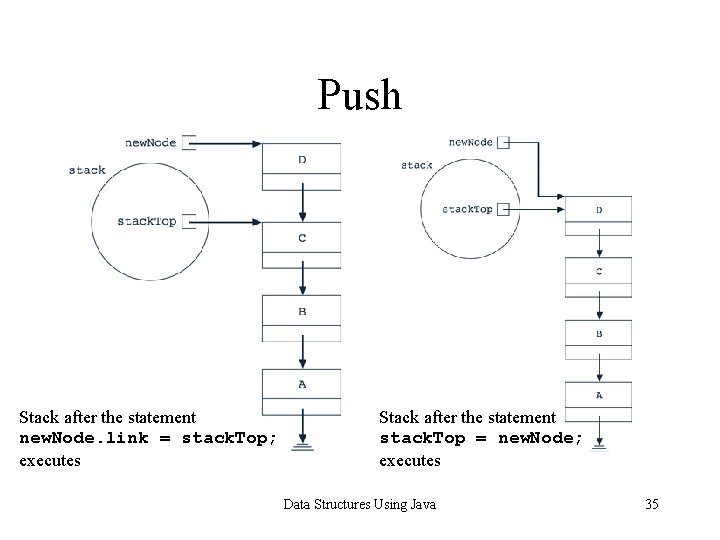Push Stack after the statement new. Node. link = stack. Top; executes Stack after the statement stack. Top = new. Node; executes Data Structures Using Java 35Return Top Element public Data. Element top() throws Stack. Underflow. Exception { if(stack. Top == null) throw new Stack. Underflow. Exception(); return stack. Top. info. get. Copy(); }//end top Data Structures Using Java 36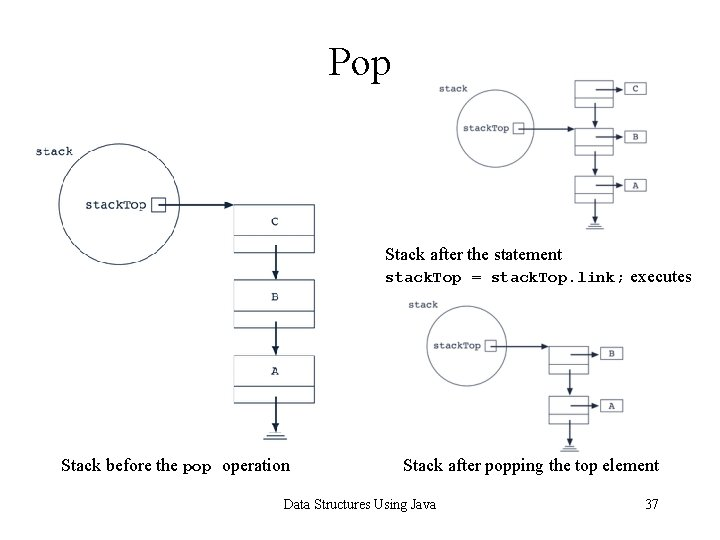Pop Stack after the statement stack. Top = stack. Top. link; executes Stack before the pop operation Stack after popping the top element Data Structures Using Java 37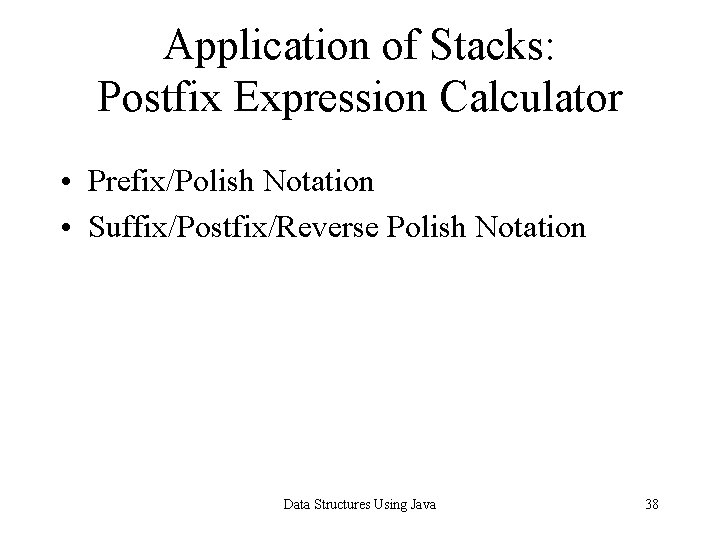Application of Stacks: Postfix Expression Calculator • Prefix/Polish Notation • Suffix/Postfix/Reverse Polish Notation Data Structures Using Java 38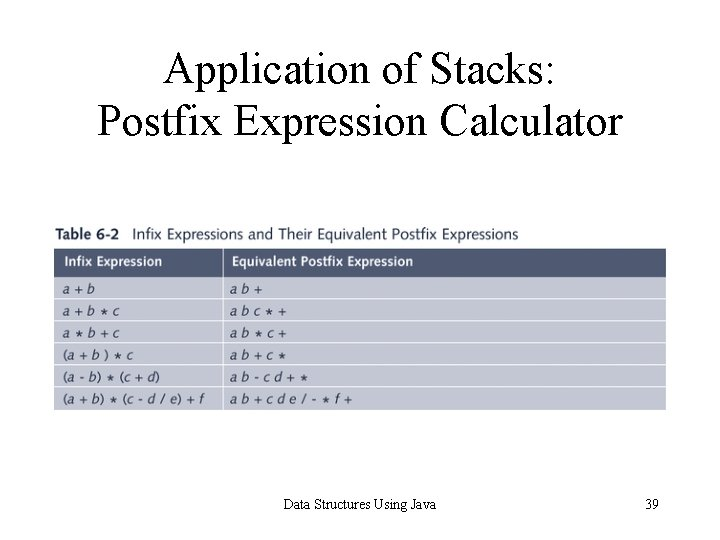Application of Stacks: Postfix Expression Calculator Data Structures Using Java 39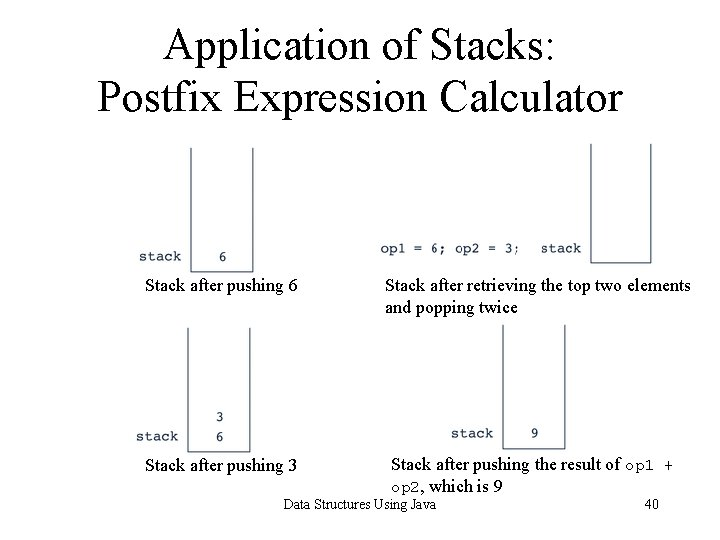Application of Stacks: Postfix Expression Calculator Stack after pushing 6 Stack after pushing 3 Stack after retrieving the top two elements and popping twice Stack after pushing the result of op 1 + op 2, which is 9 Data Structures Using Java 40Application of Stacks: Postfix Expression Calculator Stack after pushing the result of op 1 * op 2, which is 18 Stack after pushing 2 Stack after retrieving the top two elements and popping twice Stack after popping the element Data Structures Using Java 41Postfix Expression Calculator (Main Algorithm) Get the next expression while more data to process { a. initialize the stack b. process the expression c. output the result d. get the next expression } Data Structures Using Java 42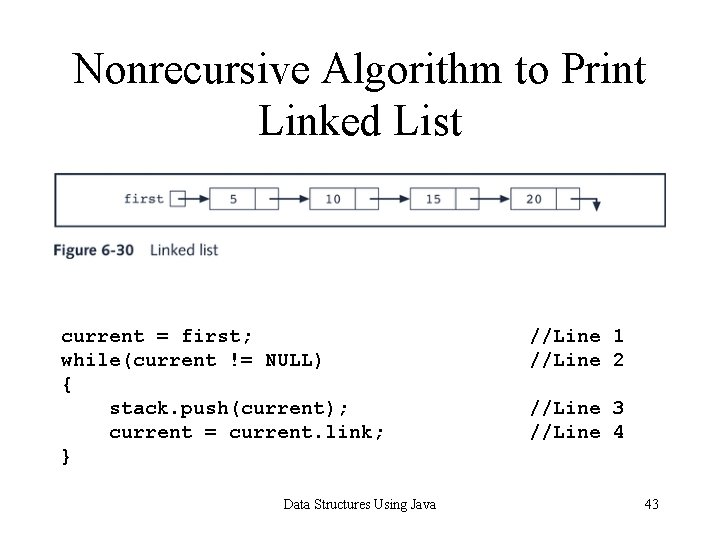Nonrecursive Algorithm to Print Linked List current = first; while(current != NULL) { stack. push(current); current = current. link; } Data Structures Using Java //Line 1 //Line 2 //Line 3 //Line 4 43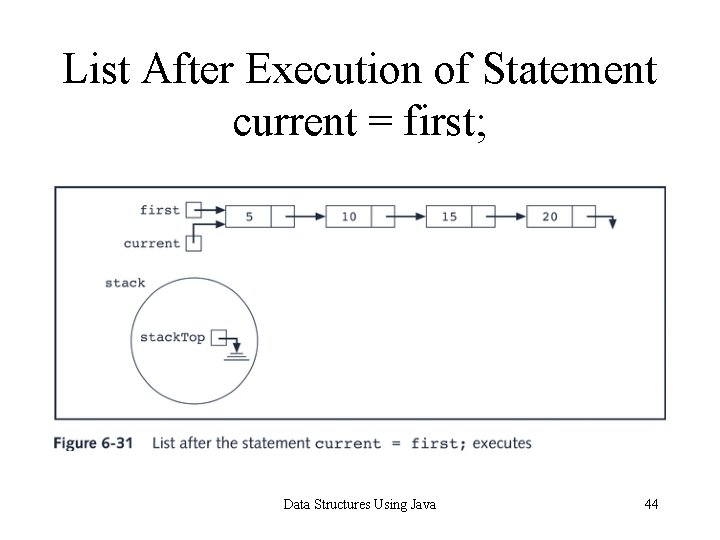List After Execution of Statement current = first; Data Structures Using Java 44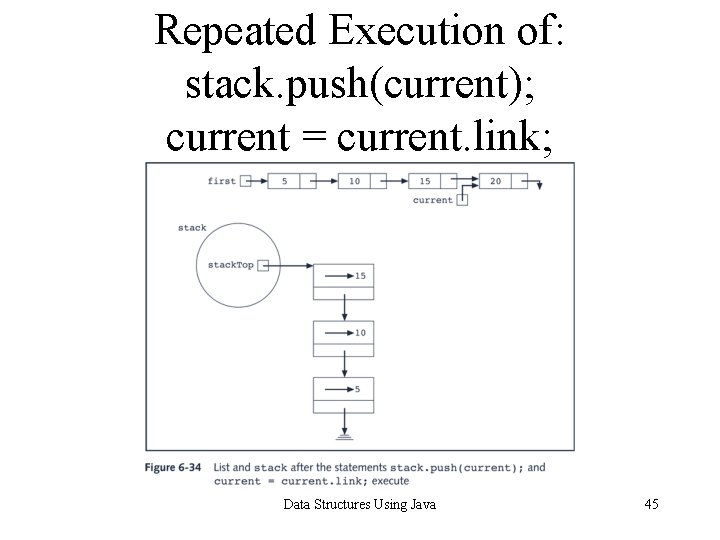Repeated Execution of: stack. push(current); current = current. link; Data Structures Using Java 45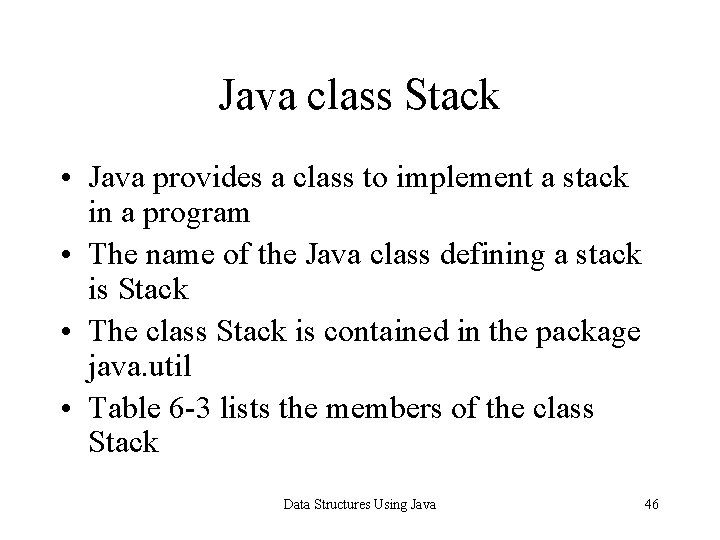Java class Stack • Java provides a class to implement a stack in a program • The name of the Java class defining a stack is Stack • The class Stack is contained in the package java. util • Table 6 -3 lists the members of the class Stack Data Structures Using Java 46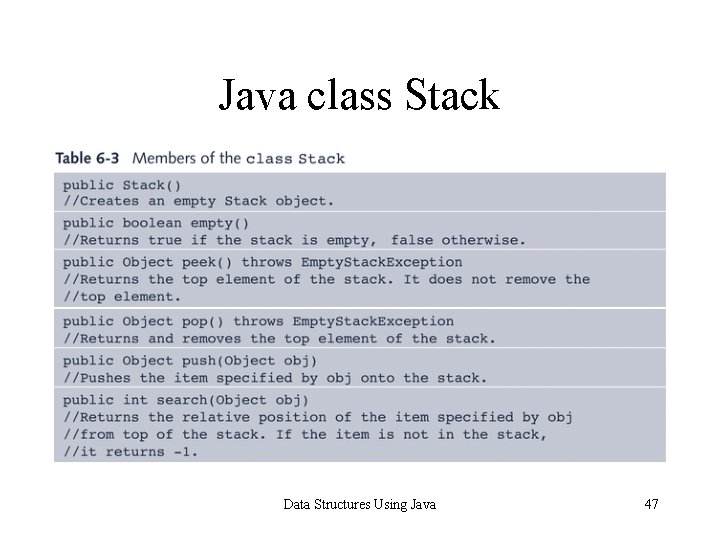Java class Stack Data Structures Using Java 47Chapter Summary • • Stack Data Structure Last In First Out (LIFO) Stacks Implemented as Arrays Stacks Implemented as Linked Lists Postfix Expression Calculator Nonrecursive Algorithm to Print Linked List Java class Stack Data Structures Using Java 48